# Finding the Percentage of a Grid that is Shaded Online Quiz

Following quiz provides Multiple Choice Questions (MCQs) related to Finding the Percentage of a Grid that is Shaded. You will have to read all the given answers and click over the correct answer. If you are not sure about the answer then you can check the answer using Show Answer button. You can use Next Quiz button to check new set of questions in the quiz.Q 1 - Finding the percentage of a grid that is shaded.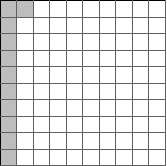### Explanation

Step 1:

We are given a 10 × 10 grid having 100 squares. The number of shaded squares gives the percentage of the grid as that is out of a total of 100 squares.

Step 2:

As the number of shaded squares is 11, the percentage of the grid here is 11%.

Q 2 - Finding the percentage of a grid that is shaded.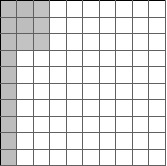### Explanation

Step 1:

We are given a 10 × 10 grid having 100 squares. The number of shaded squares gives the percentage of the grid as that is out of a total of 100 squares.

Step 2:

As the number of shaded squares is 16, the percentage of the grid here is 16%.

Q 3 - Finding the percentage of a grid that is shaded.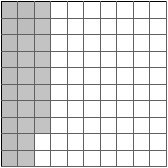### Explanation

Step 1:

We are given a 10 × 10 grid having 100 squares. The number of shaded squares gives the percentage of the grid as that is out of a total of 100 squares.

Step 2:

As the number of shaded squares is 28, the percentage of the grid here is 28%.

Q 4 - Finding the percentage of a grid that is shaded.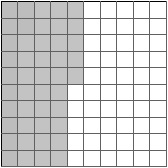### Explanation

Step 1:

We are given a 10 × 10 grid having 100 squares. The number of shaded squares gives the percentage of the grid as that is out of a total of 100 squares.

Step 2:

As the number of shaded squares is 45, the percentage of the grid here is 45%.

Q 5 - Finding the percentage of a grid that is shaded.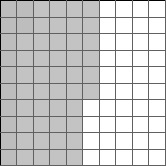### Explanation

Step 1:

We are given a 10 × 10 grid having 100 squares. The number of shaded squares gives the percentage of the grid as that is out of a total of 100 squares.

Step 2:

As the number of shaded squares is 56, the percentage of the grid here is 56%.

Q 6 - Finding the percentage of a grid that is shaded.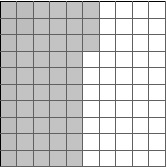### Explanation

Step 1:

We are given a 10 × 10 grid having 100 squares. The number of shaded squares gives the percentage of the grid as that is out of a total of 100 squares.

Step 2:

As the number of shaded squares is 53, the percentage of the grid here is 53%.

Q 7 - Finding the percentage of a grid that is shaded.### Explanation

Step 1:

We are given a 10 × 10 grid having 100 squares. The number of shaded squares gives the percentage of the grid as that is out of a total of 100 squares.

Step 2:

As the number of shaded squares is 42, the percentage of the grid here is 42%.

Q 8 - Finding the percentage of a grid that is shaded.### Explanation

Step 1:

We are given a 10 × 10 grid having 100 squares. The number of shaded squares gives the percentage of the grid as that is out of a total of 100 squares.

Step 2:

As the number of shaded squares is 30, the percentage of the grid here is 30%.

Q 9 - Finding the percentage of a grid that is shaded.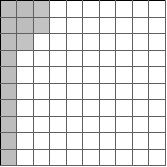### Explanation

Step 1:

We are given a 10 × 10 grid having 100 squares. The number of shaded squares gives the percentage of the grid as that is out of a total of 100 squares.

Step 2:

As the number of shaded squares is 15, the percentage of the grid here is 15%.

Q 10 - Finding the percentage of a grid that is shaded.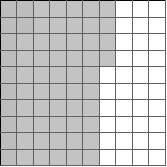### Explanation

Step 1:

We are given a 10 × 10 grid having 100 squares. The number of shaded squares gives the percentage of the grid as that is out of a total of 100 squares.

Step 2:

As the number of shaded squares is 64, the percentage of the grid here is 64%.